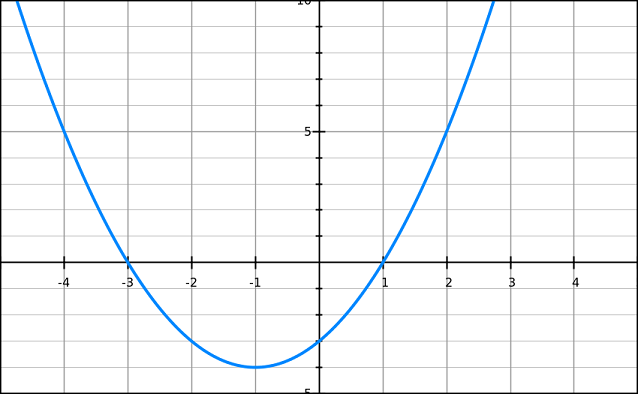# What is a parabola?

### Visual example

The following image shows the shape of a parabola. Said another way, the curve is parabolic.### Algebraic Form

An equation that graphs a parabola takes the following form:

$$y=Ax^2+Bx+C$$

A quadratic equation has the shape of a parabola when graphed.

### Properties of a parabola

A parabola extends to infinity in direction, but does not extend to infinity in the other direction -- it has the shape of a U. The point right at the tip of the parabola is called the vertex. It also has a focus (a single point inside the parabola) and a directrix (a line perpendicular to the parabola). The parabola consists of all points equidistant from the focus and the directrix.